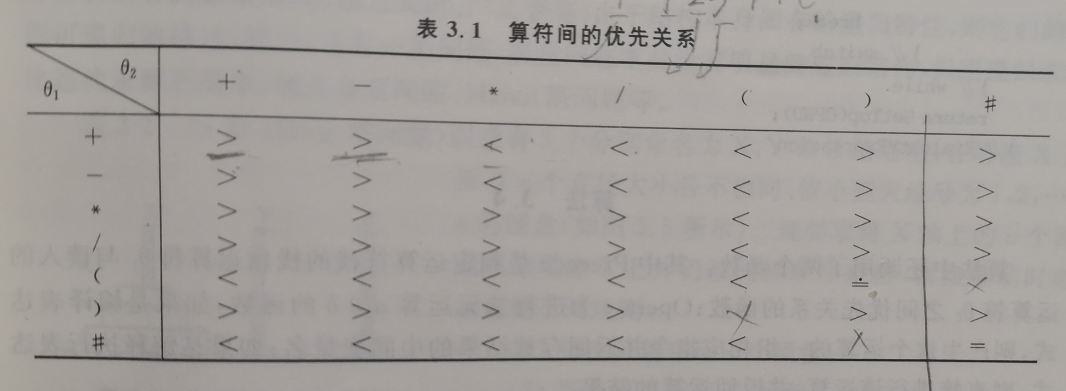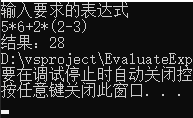## 栈的实际运用

### 使用栈来计算表达式

1. 优先级 先乘除 后加减
2. 从左到右
3. 先算括号里面的

#### 核心思路

##### 优先关系1. θ1 < θ2 θ1 优先级低于θ2
2. θ12 θ1 优先级高于θ2
3. θ12 θ1 优先级等于θ2

θ1 表示右运算符， θ2 表示左运算符

1. 从左到右 ：一般从我们人类的角度思考，计算从左到右，这一条往往是直接刻在我们潜意识里的，但是对于计算机来说，这一点得我们告诉ta。

简单概括一下**，同一个运算符也分左右，右运算符大于左运算符（相对操作数左右而言）**。

2. 先计算括号里面的，后计算括号外面的，这一句，可以表示为 ，右运算符的右括号比任何运算符优先级都底遇到左运算符的左括号优先级相等

那么= 之时就是，去括号之时

3. 为了能够正确的结束表达式的运算，我们还需加入 # 作为休止符，当然他也成对，表达式开始一个，结尾一个。

4. 当然这个表空缺的地方 表示 表达式错误

##### 运算符之间的pk

#1+3*5 #

#### 算法描述

OperandType EvaluaEXpression()
{
InitStack(OPTR);
Push(OPTR);//作为开头标志
InitStack(OPND); c=gatchar();
while(c!=#||GetTop!=#)
{
if(In(c,op))//不是操作符进操作数栈
{
Push(OPND,c);
c=getchar();
}
else{
switch(Precode(GetTop(OPTR),c))
{
case '<'://栈顶元素优先级比较低
Push(OPTR,c);
break;
case '='://去括号
Pop(OPTR,x);
break;
case '>':
Pop(OPTR,theta);
Pop(OPND,a);
Pop(OPND,b);
Push(OPND,Operator(b,theta,a));
break;
}//switch
}//else
}//while
return Getop(OPND);
}


### 代码

#### 运行结果#### 核心代码

#include"EvaluateExpression.h"
#include"Stack.h"
int IsOperator(char c)
{
switch (c)
{
case '+':return 0; break;
case '-':return 1; break;
case '*':return 2; break;
case '/':return 3; break;
case '(':return 4; break;
case ')':return 5; break;
case '#':return 6; break;
}
return -1;
}
/* 运算符对照表 */
// >  <  =   $表示错误 const char Prior = { {'>','>','<','<','<','>','>'},//+ {'>','>','<','<','<','>','>'},//- {'>','>','>','>','<','>','>'},//* {'>','>','>','>','<','>','>'},// / {'<','<','<','<','<','=','$'},//(
{'>','>','>','>','$','>','>'},//) {'<','<','<','<','<','$','='}// #
};

char OperatorPrior(int c1, int c2)
{
return Prior[c1][c2];
}

int calculate(int num1, int c, int num2)
{
switch (c)
{
case 0:return num1 + num2;break;
case 1:return num1 - num2;break;
case 2:return num1 * num2;break;
case 3:return num1 / num2; break;
}
return 0;
}

int EvaluateExpression(std::string s)
{
SqStack OPTR, OPND;
if (!Init_SqStack(OPND) || !Init_SqStack(OPTR))
exit(0);
Push(OPTR, 6); //插入开始标志 方便判断计算是否完成
s = s + '#'; //判读是否结束
char c = s;
int i = 1;
int e,b,a;
int tmp;
GetTop(OPTR, e);
while (c!='#'|| e!=6) {  //表达式未读完或者运算未完
int sum = 0;
if (c >= '0' && c <= '9') {
while (c >= '0' && c <= '9') {  //输入高位数
sum = sum * 10 + (c - '0');
c = s[i++];
}
Push(OPND,sum);  // 把读进的数字入数字栈
}
else {
tmp=IsOperator(c);
switch (OperatorPrior(e, tmp))
{
case '<':  //栈顶元素优先权低
Push(OPTR, tmp);
c = s[i++];
break;
case '=':
Pop(OPTR,e);  // 脱括号
c = s[i++];
break;
case '>':  //退栈并将运算结果入栈
Pop(OPTR, e);
Pop(OPND, a);
Pop(OPND, b);
Push(OPND, calculate(b, e, a));
break;
}
}
GetTop(OPTR, e);//读取当前运算判断是否为 #
}
GetTop(OPND, e);
return e;
}